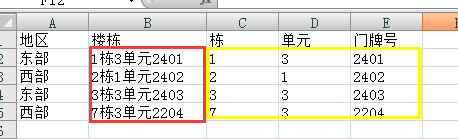python 提取数据到新列,用pandas3个回答

`````` import pandas as pd
import re

df = pd.read_excel("Q696322.xlsx", sheet_name = 0)
df['栋'] = df.apply(lambda x: re.match("\\d+(?=栋)", x["楼栋"], re.M|re.I).group(), axis=1)
df['单元'] = df.apply(lambda x: re.match("\\d+[^\\d]+(\\d+)", x["楼栋"], re.M|re.I).group(1), axis=1)
df['门牌号'] = df.apply(lambda x: re.match("\\d+[^\\d]+\\d+[^\\d]+(\\d+)", x["楼栋"], re.M|re.I).group(1), axis=1)
df.to_excel("Q696322_result.xlsx")
``````

`之前还回答了几个问题，如果问题解决，麻烦一并采纳，谢谢`darklinboxs 好吧，如果是这几列写到另外一个新的表改怎么做呢？darklinboxs 大佬，你的列是怎么定位的比如我要填过几列写入，栋，单元，门牌号这几列。

`````` Sub main()
iRowFirst = 2     ' 数据第一行
iRowLast = 5      ' 数据最后一行
iColStr = 2       ' 楼栋字符串
iColBuild = 3     ' 栋
iColUnit = 4      ' 单元
iColHouse = 5     ' 门牌号

For i = iRowFirst To iRowLast
strstr = Sheet1.Cells(i, iColStr)
Sheet1.Cells(i, iColBuild) = Mid(strstr, 1, 1)
Sheet1.Cells(i, iColUnit) = Mid(strstr, 3, 1)
Sheet1.Cells(i, iColHouse) = Mid(strstr, 6)
Next
End Sub

``````pandas dataframe数据提取的方法
pandas dataframe数据提取
pandas系列学习（四）：数据提取

Python Pandas修改列类型

Python数据扩展包之Pandas

pandas数据新索引：reindex
DataFrame.reindex(labels=None, index=None, columns=None, axis=None, method=None, copy=True, level=None, fill_value=nan, limit=None, tolerance=None) 常用关键参数： method：插值填充方法 fill_value：引入缺失数据值 columns
Pandas之获取行和列的数据
Python数据分析是Python领域中的高阶课程，也是Python程序员熟练掌握Python的必学技能，学会此系列课程之后，就能够在纷繁杂乱的数据中分析出自己想要的数据。
pandas 快速添加行和列数据

pandas 某列数据筛选
#将某一行的编码格式设置为 utf-8,支持中文显示和查找 stock_data['name'] = [i.encode('utf8') for i in stock_data['name']]#判断一列中的字符信息，并删除改行 for index,row in stock_data.iterrows(): if '退市'in row['name']: stock_da...
Pandas如何将Series的复合索引提取为列？

Python用pandas处理csv数据

pandas通过loc生成新的列
pandas中一个很便捷的使用方法通过loc、iloc、ix等索引方式，这里记录一下： df.loc[条件,新增列] = 赋初始值 如果新增列名为已有列名，则在原来的数据列上改变 import pandas as pd import numpy as np data = pd.DataFrame(np.random.randint(0,100,40).reshape(10,4),colu...
pandas切割字符串并保存为新列

python 提取文件指定列

python pandas常用数据处理方法
pandas 1、header = 0 不同于 header = None header = 0 表示 第0行为列 header = None 表示读取的时候 认为没有标题，全是数据 可以用 skiprows = 1 跳过列名 2、pandas 获取指定的行列数据 df.iloc[0:2,[0,3]] #读取 第 [0,2)行的第[0,3)列 3、创建 df = DataFr...
python pandas 处理日期数据

python pandas 的字符数据的处理方法

Python 之 Pandas (二)选择数据

Python 之 Pandas (三)选择数据

python之pandas使用：数据的选择

python的pandas处理数据第一次

python 提取sqlit数据

python导入数据与提取数据

python　爬虫　数据提取

Python提取数据
Python提取数据python提取数据 目前实现的代码：import redef read_write_file(in_file_path, out_file_path, reg_expression, line_per_loop): in_file = open(in_file_path) out_file = open(out_file_path, "a") #pat
Python之数据提取

python——数据提取，处理
#读入表 import pandas as pd urls = pd.read_csv(&quot;/home/kong/Desktop/url.csv&quot;) #print(urls.shape)　　　　#输出表的大小 nrow = urls.shape #输出行数　同理，列数——shape print(nrow) print(urls.values) #输出指定...
python数据提取方法
# json #数据交换格式,看起来像python格式(字典、列表）类型的字符串 使用前需要import json 哪里会返回json数据？ 1.浏览器切换到手机版 2.抓包app json.loads #把json字符串转化成python类型 json.loads(json字符串） json.dumps # 把python 类型转化为json字符串 json.du...
Python:提取网页数据

Pandas应用于数据提取的一些小知识
import pandas as pddf = pd.read_csv( )# 默认显示列表数据的前5行df.head( )
pandas wind 数据提取简单demo
#!/usr/bin/env python #coding:utf-8 from WindPy import w from datetime import datetime from pandas import Series from pandas import DataFrame import glob import os import pdb import csv import sys de

Pandas库 http://pandas.pydata.org Pandas是Python第三方库，提供高性能易用数据类型和分析工具。 import pandas as pd 数据类型：Series, DataFrame 基于数据类型的各类操作：基本操作、运算操作、特征类操作、关联类操作 Numpy:     基础数据类型：ndarray;
python pandas用均值去填充该行/列的空值

python | pandas 改变列的位置、填充缺失值

python : pandas 画移动平均线 新的代码
python 3.7 , pandas 0.23 以上 数据文件 660001.csv 内容格式: 日期，基金净值，累计净值 date,jz,ljjz 2016-01-04,2.4990,3.0990 2016-01-05,2.4277,3.0277 ... ...  2019-02-12,1.8362,2.4362 2019-02-13,1.8589,2.4589 pd_roll_mean_i...
python 用pandas，实现贝叶斯分类

【Pandas】Pandas数据分类